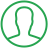# How To Import Math In Python

Last Updated : Oct 16, 2023In this article we will show you the solution of how to import math in python, the Python Math Module is covered in this article. Using the Math module in Python, we can quickly calculate a wide range of mathematical calculations.

When handling intricate financial computations or challenging scientific activities, mathematical calculations may occasionally be necessary.

The Python math module can handle these difficult calculations.

The math module's functions can be used to do both straightforward operations like addition (+) and subtraction (-), as well as trickier ones like trigonometric and logarithmic ones.

We will now discuss the idea of how to import math functions in python with an example.

## Step By Step Guide On How To Import Math In Python :-

```# Python program to show how to use the exp() function.
# importing the math module
import math
# declaring some value
num1 = 4
num2 = -3
num3 = 0.00
# passing above values to the exp() function
print( f"The exponential value of {num1} is: ", math.exp(num1) )
print( f"The exponential value of {num2} is: ", math.exp(num2) )
print( f"The exponential value of {num3} is: ", math.exp(num3) )```
1. Since the exp() function will be utilized in this program, the math module is imported.
2. To indicate the three numbers for which we want to calculate the exponential value, we define three variables: num1, num2, and num3.
3. The math module's exp() function receives these integers and outputs the results using the print() method.
4. The values of num1, num2, and num3 are dynamically inserted into the print statement using the f-string.
5. The results show the exponential function values for 1, 2, and 3.

## Conclusion :-

As a result, we have successfully learned how to import math functions in python with an example.

So in this tutorial, we have talked about Python's math module. Numerous functions in the math module can be used to carry out mathematical operations.

Each function in the math module is discussed, and we also share part of the function's program codes.

I hope this article on how to import math in python helps you and the steps and method mentioned above are easy to follow and implement.

##About Dikshita

Passionate Electronics and Communication Engineering student with expertise in web development (HTML, CSS, JS,PHP, Bootstrap, React.js) and content writing. Eager problem solver and tech enthusiast, adept at creating engaging web experiences.